This tutorial demonstrates how to use the YEARFRAC Function in Excel, Google Sheets, and VBA to return the fraction of the year between dates.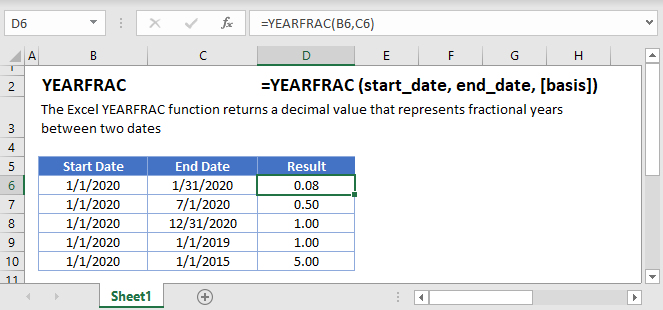## YEARFRAC Function Overview

The YEARFRAC Function Returns the fraction of a year between two dates.

To use the YEARFRAC function, select a cell and type: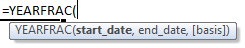(Notice how the formula input appear)

## YEARFRAC Function Syntax and Inputs:

start_date – The start date

end_date – The end date

[basis] – [Optional] The basis that determines the number of days in the year.

## YEARFRAC Examples

### Less Than One Year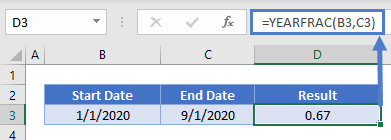Whole Year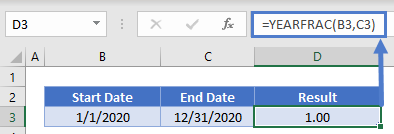More than One Year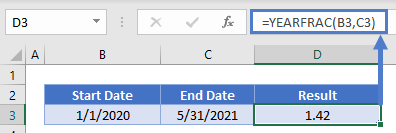## YEARFRAC Basis

By default, Excel uses the US 30/360 basis, where a year is 360 days long and each month has 30 days. Instead you can change the basis: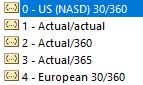Here is the impact of changing the basis: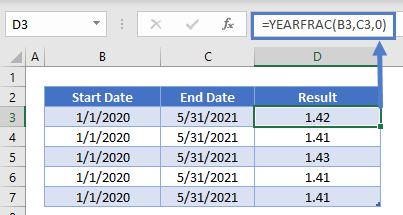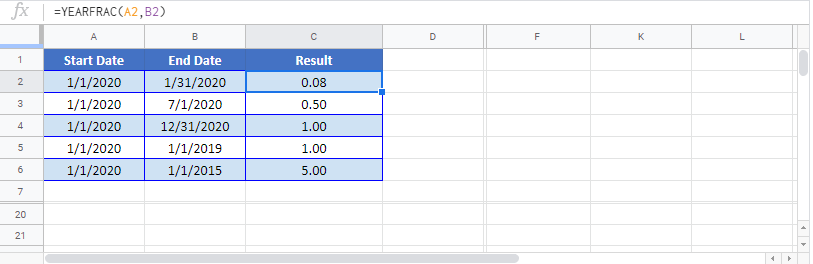`application.worksheetfunction.yearfrac(start_date,end_date,basis)`